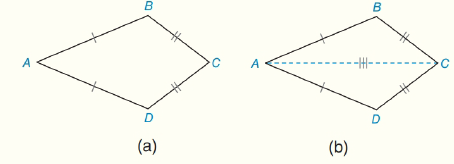Chapter 4.CT, Problem 15CTElementary Geometry For College St...

7th Edition
Alexander + 2 others
ISBN: 9781337614085

Solutions

Chapter
SectionElementary Geometry For College St...

7th Edition
Alexander + 2 others
ISBN: 9781337614085
Textbook Problem

Complete the proof of the following theorem:“In a kite, one pair of opposite angles are congruent.”Given: Kite ABCD; A B ¯ ≅ A D ¯   and  B C ¯ ≅ D C ¯ Prove: ∠ B ≅ ∠ DPROOF Statements Reasons 1_________2. Draw A C ¯ 3.__________4. Δ A C D ≅ Δ A C B 5.__________ 1.______________2. Through two points, there is exactly one line.3. Identity4.____________5._________________

To determine

To Find:

The proof of BD

Explanation

Given:

Kite ABCD,

Approach:

Consider the theorem,

“In a kite, one pair of opposite angles are congruent.”

 PROOF Statements Reasons 1. AB¯≅AD¯ and BC¯≅DC¯2. Draw AC¯3. AC¯≅AC¯4. ΔACD≅ΔACB5

Still sussing out bartleby?

Check out a sample textbook solution.

See a sample solution

The Solution to Your Study Problems

Bartleby provides explanations to thousands of textbook problems written by our experts, many with advanced degrees!

Get Started

Finding Inverse Functions Find the inverse function of f. 56. f(x)=3xx2

Precalculus: Mathematics for Calculus (Standalone Book)

Sketch the graphs of the equations in Exercises 512. 2y+x=1

Finite Mathematics and Applied Calculus (MindTap Course List)

Evaluate ∫16t3 ln t dt. 4t4 + C 4t3 ln t − 16t3 + C 4t4 − t3 ln t + C 4t4 ln t − t4 + C

Study Guide for Stewart's Single Variable Calculus: Early Transcendentals, 8th